Mobile QR Code1. (Department of Electronic Engineering, Sogang University, Seoul 04107, Korea)
2. (Samsung Electronics Company, Limited, Hwaseong 18448, Korea)

Analog-to-digital converter (ADC), non-binary digital-to-analog converter (DAC), partially monotonic switching, redundancy, successive-approximation register (SAR)

## I. INTRODUCTION

Recently, as wireless local area network (WLAN) services have become more commonplace with the rise of mobile communication technologies such as 5G networks [1,2], the demand for related mobile communication systems has increased explosively. For mobile devices of such communication systems, analog-to-digital converters (ADCs) that have a small area with excellent power efficiency and operate at a speed of exceeding 100-MS/s with a resolution of over 10-bit are essential [3-6]. Among various ADC architectures to satisfy the resolution and operating speed required by communication systems, the competitiveness of successive-approximation resistor (SAR) ADCs to meet low-power small-area specifications is increasing. In addition, SAR ADCs have the advantage of maintaining excellent performance in terms of power consumption even if the speed is adjusted as needed. However, SAR ADCs have a limitation in their operating speed due to the residual voltage settling time of the digital-to-analog converter (DAC) [7,8]. To solve this problem, the multi-channel time-interleaving method to connect several identical sub-ADCs of low operating speed in parallel or the 2b/cycle method to output a 2-bit digital code in one cycle is widely used. However, these techniques require additional calibration circuits that increase power and area [9,10].

This work proposes an 11-bit SAR ADC with an operating speed of 160-MS/s based on a non-binary DAC for settling error correction. High-speed operation is facilitated by correcting settling errors through the redundancy of non-binary DAC, and power consumption is reduced by applying the partially monotonic switching scheme.

This work is organized as follows. Section II describes the overall structure of the proposed SAR ADC, and Section III describes each of the proposed circuit design techniques in detail. Section IV summarizes the measurement results, and the paper concludes with Section V.

## II. PROPOSED SAR ADC ARCHITECTURE

An 11-bit 160-MS/s single-channel SAR ADC proposed in this paper is shown in Fig. 1. The proposed ADC consists of a non-binary DAC with redundancy, a comparator, a SAR logic, and a non-binary to binary conversion logic.

The non-binary DAC shown in Fig. 1 is composed of a 2-bit capacitor-array (C-array) for a split-capacitor switching scheme and a 9-bit C-array for a monotonic switching scheme. Here, the C$_{\mathrm{U}}$, the unit capacitor of C-array, is determined to capacitance of 3.5 fF by considering the settling time and kT/C noise requirements. The most significant 8-bit output codes are decided by the upper 8-bit non-binary-weighted capacitors while the least significant 3-bit output codes are decided by the remaining three unit capacitors and the binary-weighted resistor string (R-string). In addition, bootstrapping switches are used to sample the input signal to achieve more than 11-bit linearity. The bottom plate input sampling is employed to alleviate the error resulting from the charge injection of the input sampling switches.

To minimize the dynamic offset problem caused by the common mode voltage (VCM) variation of the DAC output and reduce the average switching power consumption of the DAC, a partially monotonic switching scheme is proposed. In the proposed switching scheme, the most significant 2 bits are determined through the split-capacitor switching scheme while the remaining 9 bits are determined through the monotonic switching scheme.

In the proposed non-binary DAC, redundancy is applied to correct incomplete settling errors as follows. 64C$_{\mathrm{U}}$s are used in the capacitor with the weight of the first bit of the output code, and 34C$_{\mathrm{U}}$s are used in the capacitor with the weight of the second bit of the output code. Since 64C$_{\mathrm{U}}$s are less than twice that of 34C$_{\mathrm{U}}$s, the difference between the two capacitors, 4C$_{\mathrm{U}}$s, are used as redundant capacitors. In the same way, redundant capacitors in the subsequent decisions are determined by using the upper capacitor value smaller than twice that of the lower capacitor. As shown in Fig. 2, overlap decision range is achieved by redundant capacitors. The total redundancy resulting from the overlap decision range in the most significant bit is calculated as a total of 160~LSB.

##### Fig. 1. Proposed 11-bit 160-MS/s SAR ADC.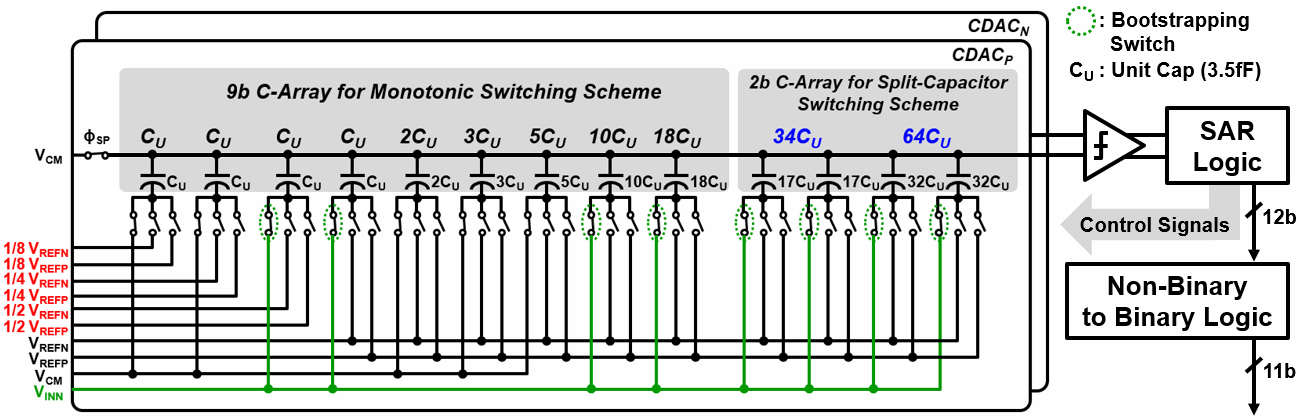##### Fig. 2. Overlapped decision range of a non-binary topology.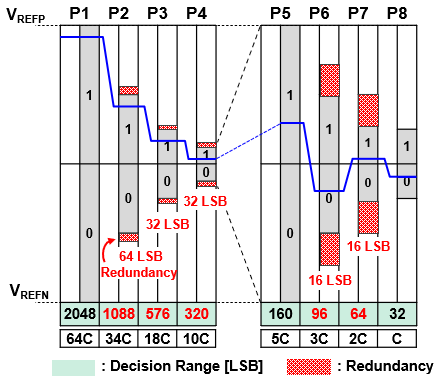## III. CIRCUIT IMPLEMENTATION

### 1. Non-binary DAC with Redundancy

The proposed non-binary DAC with redundancy is shown in Fig. 3. The required residual voltage settling time of each capacitor switching considering redundancy can be calculated as in . Unlike the residual voltage of a conventional DAC with a binary weight that should be settled within 1/2LSB at an ideal output voltage without incomplete settling error, the settling range of the proposed DAC with non-binary weight has a value proportional to the redundancy range. The settling time requirement of the binary DAC (t$_{\mathrm{s,b}}$) is expressed as

##### (1)
$t_{s,b}\leq ln\left(2\times weight\right)\times \tau$

and of the non-binary DAC (t$_{\mathrm{s,nb}}$) is expressed as

##### (2)
$t_{s,nb}\leq ln\left(weight/redundancy\right)\times \tau$

where ${\tau}$ is the time constant of the DAC. As shown in Fig. 4, the maximum required settling time of the residual voltage in the conventional DAC is 7.62${\tau}$ but is 4.16${\tau}$ in the proposed DAC. In addition, the total settling time of the residual voltage of the conventional DAC and the proposed DAC are 76.2${\tau}$ and 45.8${\tau}$, respectively. As a result, the total settling time of the proposed DAC is reduced by 40\% compared to the conventional DAC. In other words, the proposed DAC can facilitate high-speed operation by relaxing the residual voltage total settling time requirements.

##### Fig. 3. Proposed non-binary DAC with redundancy.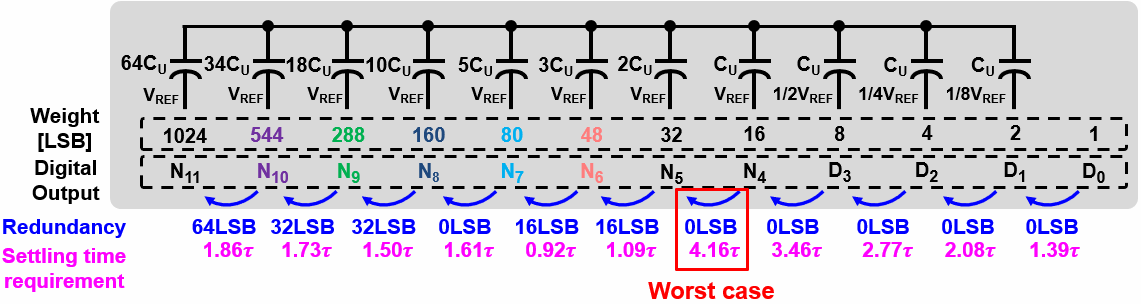##### Fig. 4. Required settling time comparison of the conventional binary DAC and the proposed non-binary DAC.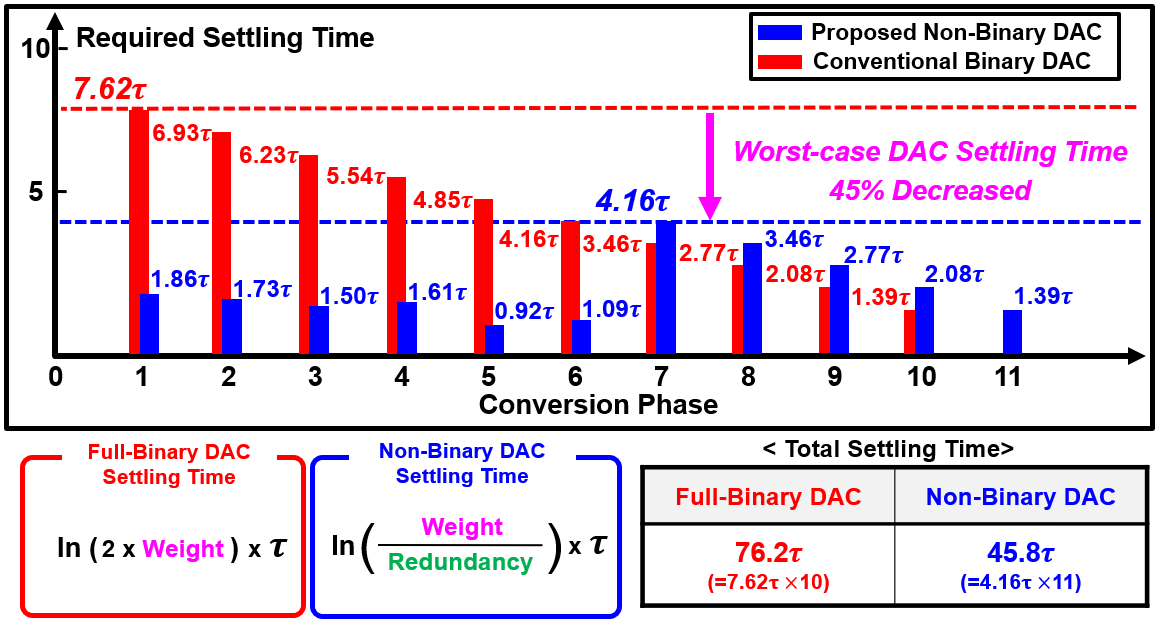### 2. Non-binary to Binary Code Encoder

To implement an encoder that converts a non-binary digital code into a binary digital code, a non-binary weight must be implemented as a combination of binary weight values. The operating principle of the implemented ADC encoder is shown in Fig. 5. The encoder consists of five simple adders. And after decomposing the non-binary weights into binary weights, the codes with the same weights are added together to output the binary code. In addition, -80 is added in 2's complement form (‘11110110000’) to prevent overflow caused by the extra weight for redundancy.

##### Fig. 5. Non-binary to binary code conversion.### 3. Low-power Double-tail Dynamic Comparator

A low-power double-tail comparator shown in Fig. 6 is employed . It consists of two stages: a dynamic pre-amplifier input stage and a latch stage which is driven by positive feedback. The comparator operates as follows. During the reset phase of the comparator outputs, when CLK goes low, the drain nodes of PMOS M4 and M5 are pre-charged to VDD, the drain nodes of NMOS M6 and M9 are discharged to VSS, and the outputs are reset to high. During the comparison phase, when CLK goes high, NMOS M1 and PMOS M12 are turned on, and PMOS M4 and M5 are turned off. Then voltages of the TN and TP are discharged according to the differential input signal and are applied to the input of the next latch stage. The voltage difference between the TN and TP causes a difference in the amount of current flowing through M7 and M8. And positive feedback in the latch stage reduces the regeneration delay.

##### Fig. 6. Low-power double-tail dynamic comparator.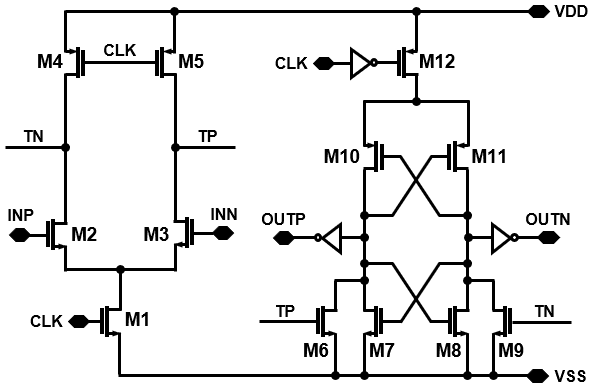### 4. Partially Monotonic Switching Scheme

A partially monotonic switching scheme that determines the most significant 2 bits using the split-capacitor switching scheme and the remaining 9 bits using the monotonic switching scheme is proposed. Fig. 7 shows the DAC output voltages of the conventional 11-bit SAR ADC with the proposed switching scheme when the output code is '10000000000'. The upper 2-bit decision changes V$_{\mathrm{OUTP}}$ and V$_{\mathrm{OUTN}}$, and the subsequent 9-bit decision changes only V$_{\mathrm{OUTP}}$, where V$_{\mathrm{OUTP}}$ and V$_{\mathrm{OUTN}}$ are positive and negative DAC output voltages respectively.

Fig. 8 shows the VCM variation of the DAC output in two cases of using the proposed switching and the monotonic switching. The VCM variation of the DAC causes a dynamic offset of the comparator, resulting in linearity degradation of the SAR ADC . The proposed switching scheme reduces the maximum VCM variation of the DAC output by 87.5\% since there is no VCM variation when deciding the most significant 2 bits. By using the proposed switching scheme, the dynamic offset can be minimized by ensuring that the VCM variation of the DAC output is less than 1/16 of the reference voltage (V$_{\mathrm{REF}}$).

The average switching power consumption of the proposed partially monotonic switching scheme is

##### (3)

$E_{avg,proposed}=\sum _{i=1}^{k}\left(2^{n-1-2i}\right)\left(2^{i}-1\right)\left(C/2\right){V_{REF}}^{2}$

$+\sum _{l=k+1}^{n-1}\left(2^{n-2-l}\right)C{V_{REF}}^{2}$

$+\left(2^{n-4-k}\right)\times \left(2-\frac{1}{2^{k-1}}\right)C{V_{REF}}^{2}.$

In (3), the first and second terms present the average switching power consumption in the split-capacitor switching and in the monotonic switching, respectively [16,17]. The third term is the average switching power consumption when converting from split-capacitor switching to monotonic switching. Here, n is the resolution of the ADC and k is the number of bits using split-capacitor switching scheme. Table 1 compares the maximum VCM variation and average switching power consumption of the DAC output of three switching schemes: monotonic switching scheme, split-capacitor switching scheme, and proposed switching scheme.

##### Fig. 7. SAR operation with the proposed switching scheme.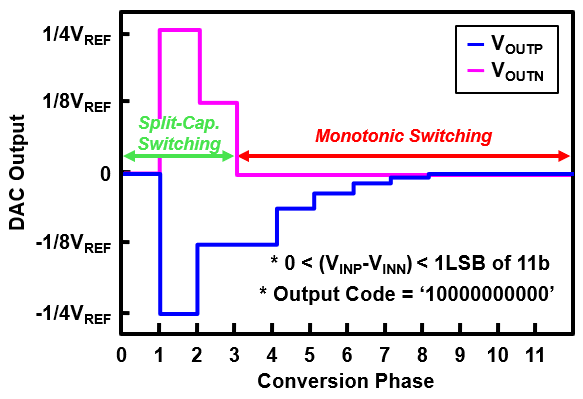##### Fig. 8. VCM variations of the proposed switching-based DAC.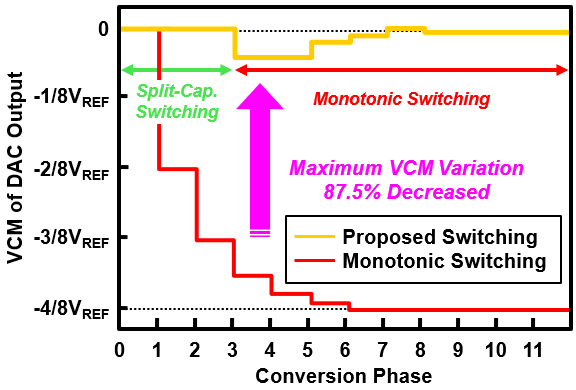##### Table 1. Performance comparison of switching schemes
 Scheme Split-Cap. Monotonic Proposed Max VCM 0 1/2 VREF 1/16 VREF Paverage 682 CVREF2 512 CVREF2 400 CVREF2 No. of CUs in DAC 2N 2N-1 2N-1

### 5. Environmental-insensitive Capacitor Layout

To reduce the impact of parasitic capacitance between adjacent capacitors, the C-Array of the proposed ADC employs an encapsulated capacitor structure as shown in Fig. 9. As shown in top views of M3 and M4, the top and bottom plate metal layers were arranged alternately to achieve the fringing capacitance. The outermost side of the capacitor is shielded as shown in the cross-sections of A-B and C-D. Such a shielded capacitor structure can minimize linearity degradation due to parasitic capacitance between adjacent capacitors by maintaining the same layout conditions for each unit capacitor while securing the required capacitance .

Fig. 10 illustrates the layout floorplan of the C-array with an area of 50.5 ${\mu}$m$\times$37.4 ${\mu}$m. In order to compensate for the effect of gradient error, the C-array layout is based on the common-centroid methods.

##### Fig. 9. Proposed environment-insensitive and 3D-encapsulated capacitor structure.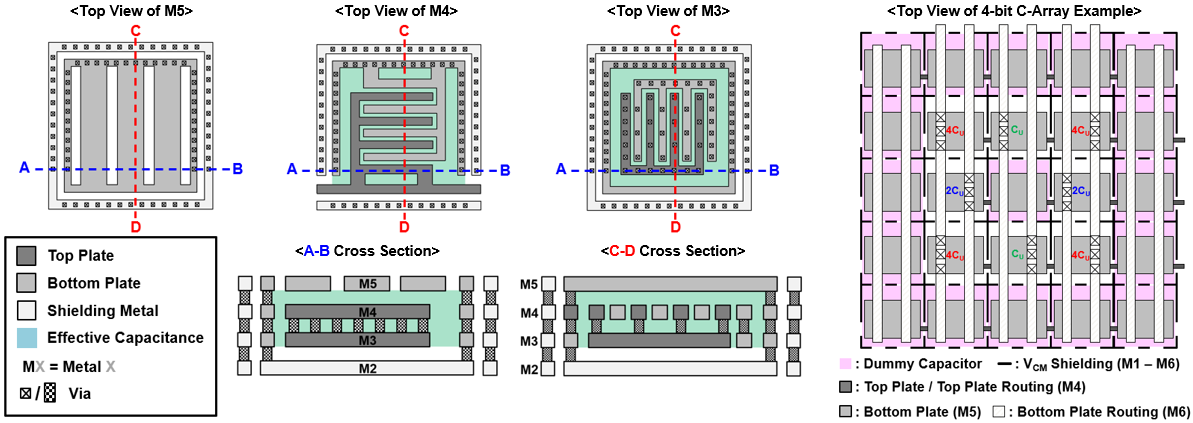##### Fig. 10. Common-centroid layout floorplan of DAC capacitors.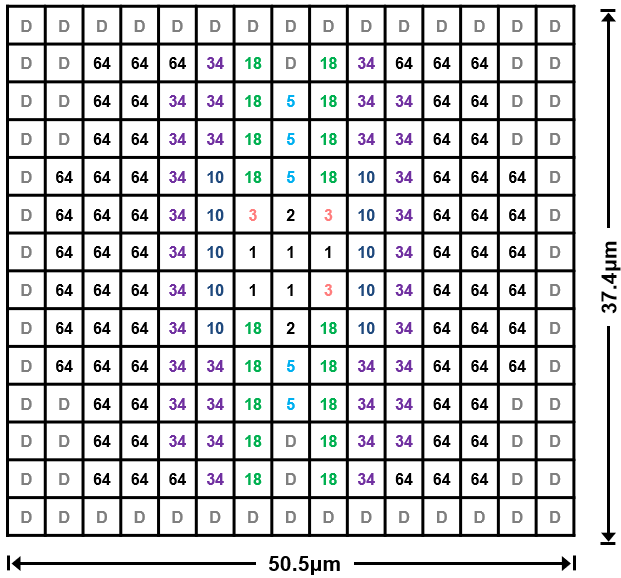## IV. MEASUREMENT RESULTS

The proposed 11-bit 160 MS/s SAR ADC based on a non-binary DAC is fabricated on a 28 nm CMOS process. Fig. 11 shows the (a) layout and (b) power distribution of the prototype ADC. The prototype ADC occupies an active die area of 0.026 mm$^{2}$ including the R-string area of 336 ${\mu}$m$^{2}$. At a 1.0 V supply voltage, a total of 1.67~mW power is consumed with an operating speed of 160 MS/s. The digital logic, comparator, and R-string consume 0.97 mW, 0.4 mW, and 0.3 mW, respectively. Fig. 12 shows the measured DNL and INL with an input signal amplitude of 0.75 V$_{\mathrm{REF}}$, which guarantees the 11-bit linearity of the input switch. The peak DNL and INL are measured as 0.93 LSB and 1.97 LSB, respectively. The static performance of the proposed ADC is somewhat limited by capacitor mismatch resulting from process gradient errors. The measured FFT spectra at 160 MS/s with a 9 MHz and a 79 MHz inputs are shown in Fig. 13 and 14. At a 9 MHz input, the measured SNDR and SFDR are 53.5 dB and 67.5 dB, respectively. At a 79~MHz input, the measured SNDR and SFDR are 50.2~dB and 66.4 dB, respectively. The measured dynamic performance is rather limited by the laid-out capacitor mismatch, the on-resistance nonlinearity of sampling switches, and the thermal noise of the comparator.

Fig. 15 shows the ADC’s dynamic performance for various sampling rates with a 9 MHz input, presenting a SFDR higher than 66.4 dB up to a 160 MS/s conversion rate. Fig. 16 shows the dynamic performance for various input frequencies at a 160 MS/s sampling rate, presenting a SNDR above 50.1 dB up to the input frequency of 100~MHz. The performance of the prototype ADC is summarized and compared with previously reported ADCs in Table 2.

##### Fig. 11. (a) Layout; (b) power distribution of the prototype ADC.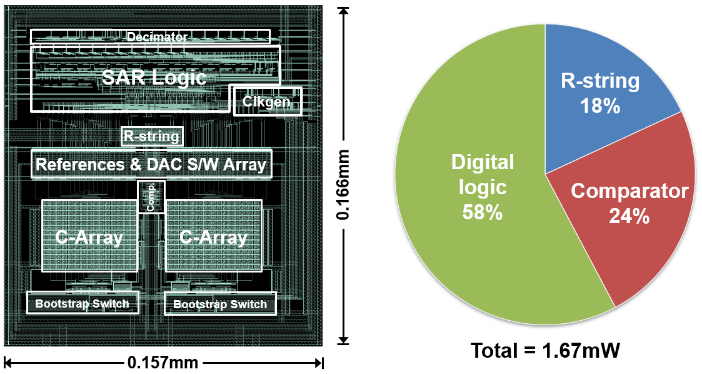##### Fig. 12. Measured static performance of the prototype ADC.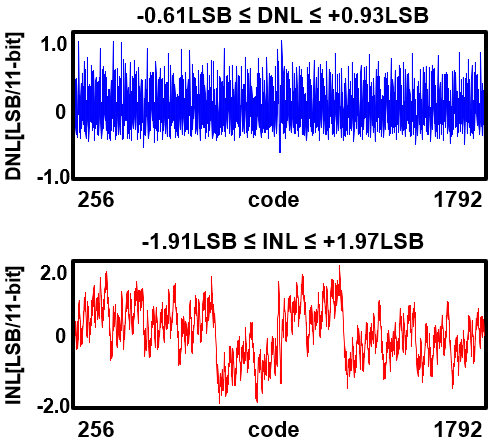##### Fig. 13. Measured FFT spectrum at 160 MS/s with a 9 MHz input.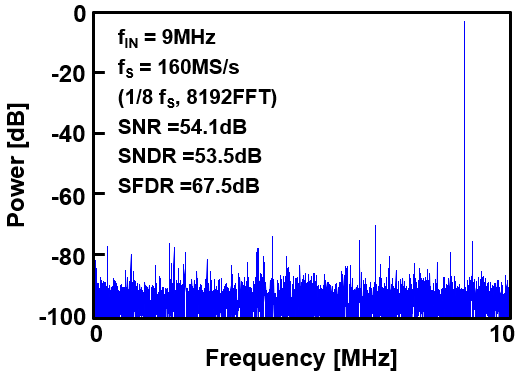##### Fig. 14. Measured FFT spectrum at 160 MS/s with a 79 MHz input.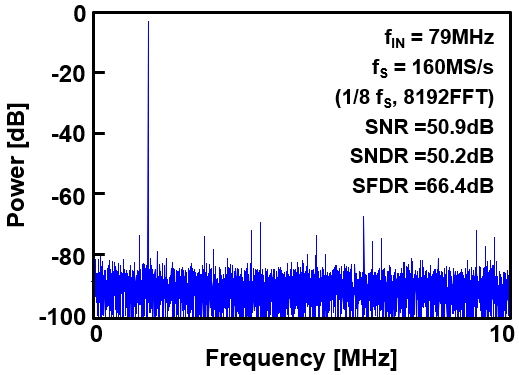##### Fig. 15. Measured SNDR and SFDR versus sampling frequency.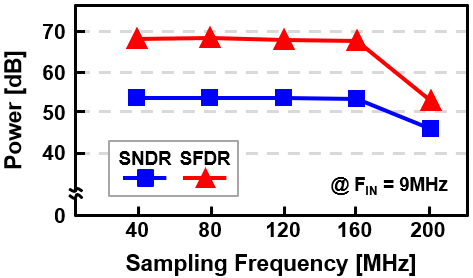##### Fig. 16. Measured SNDR and SFDR versus input frequency.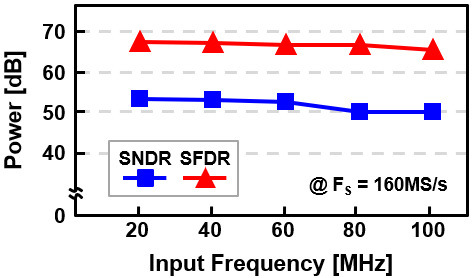##### Table 2. Performance summary and comparison of recently reported ADCs
 This work TVLSI‘17  ESSCIRC'18  CICC'19  TCASII'19  TCASII'20  ASSCC'21  Process [CMOS] 28 nm 65 nm 65 nm 28 nm 65 nm 65 nm 28 nm Resolution [bits] 11 11 12 10 12 11 12 Speed [MS/s] 160 100 125 320 100 100 120 Supply [V] 1.0 1.2 1.2 1.0 1.2 1.2 1.0 Calibration X O X X O X X DNL [LSB] 0.93/-0.61 1.0/-0.7 1.1/-0.7 0.77/-0.79 1.6/-0.8 0.91/-0.79 - INL [LSB] 1.97/-1.91 0.95/-0.8 0.75/-1.5 1.1/-0.92 1.2/-1.2 0.73/-1.01 - Peak SNDR [dB] 53.5 61.1 64.4 54.8 61.5 63.2 55.4 Peak SFDR [dB] 67.5 85.0 75.1 69.8 81.0 75.4 68.7 Power [mW] 1.67 2.4 1.7 5.5 1.9 2.1 4.7 *FoMW [fJ/conv.] 27.0 25.9 10.1 42.0 19.6 23.7 81.5 Area [mm2] 0.026 0.10 0.10 0.015 0.053 0.17 0.044 *FoMW = Power/(2ENOB·2·BW)

## V. CONCLUSION

This paper presents an 11-bit 160 MS/s Single-Channel SAR ADC. The proposed ADC employs a non-binary DAC with redundancy for the incomplete settling error correction and the partially monotonic switching scheme for low power consumption. The prototype ADC achieves a measured DNL and INL within 0.93 LSB and 1.97 LSB, respectively, with a maximum SNDR and SFDR of 53.5 dB and 67.5 dB at 160 MS/s, respectively, while consuming 1.67 mW.

## ACKNOWLEDGMENTS

This work was supported by Samsung Electronics Co., Ltd (IO201210-08008-01) and the IDEC of KAIST.

## References

1
K. Yoshioka et al., "A 0.7V 12b 160MS/s 12.8fJ/conv-step pipelined-SAR ADC in 28nm CMOS with digital amplifier technique," IEEE International Solid-State Circuits Conference (ISSCC), pp. 478-479, Feb., 2017.2
J. Park, T. An, G. Ahn, and S. Lee, "A 12.1 fJ/Conv.-Step 12b 140 MS/s 28-nm CMOS Pipelined SAR ADC Based on Energy-Efficient Switching and Shared Ring Amplifier," IEEE Trans. Circuits Syst. II, Exp. Briefs, vol. 66, no. 7, pp. 1119-1123, Jul., 2019.3
B. Verbruggen, M. Iriguchi, and J. Craninckx, "A 1.7 mW 11b 250 MS/s 2-Times Interleaved Fully Dynamic Pipelined SAR ADC in 40 nm Digital CMOS," IEEE J. Solid-State Circuits, vol. 47, no. 12, pp. 2880-2887, Dec., 2012.4
S. Wong, U. Chio, Y. Zhu, S. Sin, S. U, and R. P. Martins, "A 2.3 mW 10-bit 170 MS/s Two-Step Binary-Search Assisted Time-Interleaved SAR ADC," IEEE J. Solid-State Circuits, vol. 48, no. 8, pp. 1783-1794, Aug., 2013.5
C. Liu, C. Kuo, and Y. Lin, "A 10 bit 320 MS/s Low-Cost SAR ADC for IEEE 802.11ac Applications in 20 nm CMOS," IEEE J. Solid-State Circuits, vol. 50, no. 11, pp. 2645-2654, Nov., 2015.6
S. Sunny, Y. Chen, and C. C. Boon, "A 4.06 mW 10-bit 150 MS/s SAR ADC With 1.5-bit/cycle Operation for Medical Imaging Applications," IEEE Sensors Journal, vol. 18, no. 11, pp. 4553-4560, Jun., 2018.7
V. Tripathi and B. Murmann, "An 8-bit 450-MS/s single-bit/cycle SAR ADC in 65-nm CMOS," Proc. IEEE Eur. Solid-Stage Circuits Conf. (ESSCIRC), pp. 117-120, Oct., 2013.8
Y. Liang, R. Ding, and Z. Zhu, "A 9.1ENOB 200MS/s Asynchronous SAR ADC With Hybrid Single-Ended/Differential DAC in 55-nm CMOS for Image Sensing Signals," IEEE Sensors Journal, vol. 18, no. 17, pp. 7130-7140, Sep., 2018.9
J. Song, X. Tang, and N. Sun, "A 10-b 2b/cycle 300MS/s SAR ADC with a single differential DAC in 40nm CMOS," IEEE Custom Integrated Circuits Conference (CICC), pp. 1-4, May, 2017.10
Kang, Hyun-Wook, et al. "A Time-Interleaved 12-b 270-MS/s SAR ADC with Virtual-Timing-Reference Timing-Skew Calibration Scheme," IEEE J. of Solid-State Circuits, vol. 53, no. 9, pp. 2584-2594, Sep., 2018.11
J. G. Lim et al., “A Non-binary C-R Hybrid DAC for 12b 100MS/s CMOS SAR ADCs with Fast Residue Settling,” J. Semicoductor Technology and Science (JSTS), vol. 21, no. 4, pp. 255-262, Aug., 2021.12
H. Hong et al., "An 8.6 ENOB 900MS/s Time-Interleaved 2b/cycle SAR ADC with a 1b/cycle Reconfiguration for Resolution Enhancement," IEEE International Solid-State Circuits Conference (ISSCC) Dig. Tech. Papers, pp. 470-471, Feb., 2013.13
H. Hong et al., "A Decision-Error-Tolerant 45 nm CMOS 7b 1 GS/s Nonbinary 2b/Cycle SAR ADC," IEEE J. Solid-State Circuits, vol. 50, no. 2, pp. 543-555, Feb., 2015.14
Samaneh Babayan-Mashhadi and Reza Lotfi, "Analysis and Design of a Low-Voltage Low-Power Double-Tail Comparator," IEEE Transactions on Very Large Sacle Integration (VLSI) Systems, vol. 22, no. 2, pp. 343-352, Feb., 2014.15
L. Chen et al., " Comparator common-mode variation effects analysis and its application in SAR ADCs," IEEE International Symposium on Circuits and Systems (ISCAS), pp. 2014-2017, May., 2016.16
B. P. Ginsburg et al., “500-MS/s 5-bit ADC in 65-nm CMOS With Split Capacitor Array DAC,” IEEE J. Solid-State Circuits, vol. 42, no. 4, pp. 739-747, Apr., 2007.17
C. C. Liu, S. J. Chang, G. Y. Huang, and Y. Z Lin, “A 10-bit 50-MS/s SAR ADC With a Monotonic Capacitor Switching Procedure. IEEE J. Solid-State Circuits, vol. 45, no. 4, pp. 731-740, Apr., 2010.18
W. Kim et al., "A 0.6 V 12 b 10 MS/s Low-Noise Asynchronous SAR-Assisted Time-Interleaved SAR (SATI-SAR) ADC," IEEE J. Solid-State Circuits, vol. 51, no. 8, pp. 1826-1839, Aug., 2016.19
Y. Chung and C. W. Yen, “An 11-bit 100-MS/s Subranged-SAR ADC in 65-nm CMOS,” IEEE Transactions on Very Large Sacle Integration (VLSI) Systems, vol. 25, no. 12, pp. 3434-3443, Dec., 2017.20
S. Liu et al., “A 125 MS/s 10.4 ENOB 10.1 fJ/conv-step Multi-Comparator SAR ADC with Comparator Noise Scaling in 65nm CMOS, IEEE Eur. Solid-Stage Circuits Conf. (ESSCIRC), pp. 22-25, Sep., 2018.21
K. I. Cho et al., "A 10-b 320-MS/s Dual-Residue Pipelined SAR ADC with Binary Search Current Interpolator," IEEE Custom Integrated Circuits Conference (CICC), pp. 1-4, Apr., 2019.22
Y. H. Chung and Y. M Hsu, "A 12-Bit 100-MS/s Subrange SAR ADC With a Foreground Offset Tracking Calibration Scheme," IEEE Trans. Circuits Syst. II, Exp. Briefs, vol. 66, no. 7, pp. 1094-1098, Jul., 2019.23
J. Zhang, X. Ren, S. Liu, and C. H Chan, "An 11-bit 100-MS/s Pipelined-SAR ADC Reusing PVT-Stabilized Dynamic Comparator in 65-nm CMOS," IEEE Trans. Circuits Syst. II, Exp. Briefs, vol. 67, no. 7, pp. 1174-1178, Jul., 2020.24
S. Y. Lim et al., "An Input-buffer Embedding Dual-residue Pipelined-SAR ADC with Nonbinary Capacitive Interpolation," IEEE Asian Solid-State Circuits Conference (A-SSCC), pp. 1-3, Nov., 2021.Jae-Hyuk Lee received the B.S. degree in electronic engineering from Sogang University, Seoul, Korea, in 2020, where he is currently pursuing the Ph.D degree. His research interests include high-speed and high-resolution data converter.

Jun-Ho Boo received the B.S. degree in electronic engineering from Sogang University, Seoul, Korea, in 2017, where he is currently pursuing the Ph.D. degree. His current research interests include analog and mixed-signal circuits, data converters, and sensor interfaces.

Jun-Sang Park received the B.S., M.S., and Ph.D. degrees in electronic engineering from Sogang University, Seoul, Korea, in 2012, 2014, and 2020, respectively. He is currently with the Samsung Electronics Co., Ltd. and is developing high-speed data converter (ADC/DAC) circuits. His research interests include high-speed low-power data converter and mixed-signal circuits.

Tai-Ji An received the B.S. degree in electronic engineering from University of Seoul, Korea, in 2007, and the M.S. and Ph.D. degrees in electronic engineering from Sogang University, Korea, in 2013 and 2019, respectively. From 2007 to 2011, he was with Luxen Technologies, where he had developed various power-management and analog integrated circuits. Dr. An has been with the Samsung Electronics Co., Ltd. and is developing high-speed interface circuits and low-power high-speed high-resolution data converters.

Hee-wook Shin received the B.S. degree in electronic engineering from Jung-ang University, Seoul, Korea in 2015. He received the M.S. degrees from Sogang University, Seoul, Korea in 2017. He joined Samsung Electronics, Hwaseong, South Korea, in 2017. Currently, he is an engineer in Samsung Foundry, where he is researching the CMOS data converters and various analog front-ends.

Young-Jae Cho received the M.S. and Ph.D. degrees from Sogang University, Seoul, South Korea, in 2003 and 2007, respectively. He joined Samsung Electronics, Hwaseong, South Korea, in 2010. Currently, he is a principal engineer in Samsung Foundry, leading data converter development. His major fields are high-speed data converters and application specific analog front-ends for various applications such as digital TV, 5G network, touch controller, CIS and automotive wired/wireless communications.

Michael Choi received the M.S. and Ph.D. degrees from the University of California, Los Angeles, CA, USA, in 1998 and 2002, respectively. He joined Samsung Electronics, Hwaseong, South Korea, in 2006. Currently, he is a Master in Samsung Foundry, leading analog IP development. His expertise includes high-speed data converters and various analog front-ends for UHD digital TV, WiFi & 5G connectivity, automotive V2X, touch controller, and CMOS image sensor.

Jin-Wook Burm received the B.S. degree in physics from Seoul National University, Seoul, Korea, in 1987, the M.S. degree in physics from the University of Michigan, Ann Arbor, in 1989, and the Ph.D. degree in applied physics from Cornell University, Ithaca, NY, USA in 1995. After post-doctoral work at Cornell University and Bell Labs, Lucent Technologies, Murray Hill, NJ, he joined Dept. of Electronic Engineering, Sogang University, Seoul, Korea as an Assistant Professor in 1998, where he is now a Professor. He also worked as a Principal Scientist at Pixelplus semiconductor Inc., San Jose, CA, USA for one year starting Aug. 2006. He worked on millimeter wave ICs and high speed GaN transistors at Cornell, and optoelectronic circuits at Bell Labs. His current research interest includes high speed interface circuits and CMOS implementation of various sensors.

Gil-Cho Ahn received the B.S. and M.S. degrees in electronic engi-neering from Sogang University, Seoul, Korea, in 1994 and 1996, respectively, and the Ph.D. degree in electrical engineering from Oregon State University, Corvallis, in 2005. From 1996 to 2001, he was a Design Engineer at Samsung Electronics, Kiheung, Korea, working on mixed analog-digital integrated circuits. From 2005 to 2008, he was with Broadcom Corporation, Irvine, CA, working on AFE for digital TV. Currently, he is a Professor in the Department of Electronic Engineering, Sogang University. His research interests include high-speed, high-resolution data converters and low-voltage, low-power mixed-signal circuits design.

Seung-Hoon Lee received the B.S. and M.S. degrees in electronic engineering from Seoul National University, Korea, in 1984 and 1986, respectively, and the Ph.D. degree in electrical and computer engineering from the University of Illinois, Urbana-Champaign, in 1991. He was with Analog Devices Semiconductor, Wilmington, MA, from 1990 to 1993, as a Senior Design Engineer. Since 1993, he has been with the Department of Electronic Engineering, Sogang University, Seoul, where he is currently a distinguished research/emeritus Professor. His research interests include design and testing of high-resolution high-speed CMOS data converters, integrated sensors, and mixed-mode integrated systems.